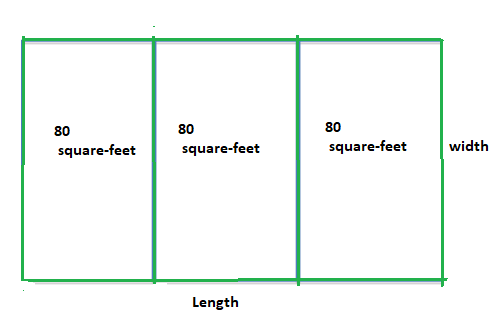# Find the dimensions of each plot.### Precalculus: Mathematics for Calcu...

6th Edition
Stewart + 5 others
Publisher: Cengage Learning
ISBN: 9780840068071### Precalculus: Mathematics for Calcu...

6th Edition
Stewart + 5 others
Publisher: Cengage Learning
ISBN: 9780840068071

#### Solutions

Chapter 1, Problem 86RE
To determine

## Find the dimensions of each plot.

Expert Solution

10×8feet

### Explanation of Solution

Given information:

A homeowner wishes to fence in three adjoining garden plots, for her children according to the figure. The area of each plot is 80squarefeet . She has 88feet 88 ft of fencing material.Calculation:

Consider the given figure,The length and width of plot be taken as fence size,

The equation for fencing would be,

4w+2l=88.....(1)wl3=80.....(2)fromequation(2)wl3=80wl=240l=240/wnowsubstitutel=240/wineqn(1)4w+2(240/w)=884w+480w88=04w288w+480=0w222w+120=0

Now we got quadratic equation now solve for width,

w=b±b24ac2a=22±2224×1×1202×1=22±4844802=22±42=22±22=11±1=10,12

Let choose

w=10putinwl=24010l=240l=24

The individual plot is one third in length of total length,

Hence, the dimensions of each plot will be 10×8feet

### Have a homework question?

Subscribe to bartleby learn! Ask subject matter experts 30 homework questions each month. Plus, you’ll have access to millions of step-by-step textbook answers!